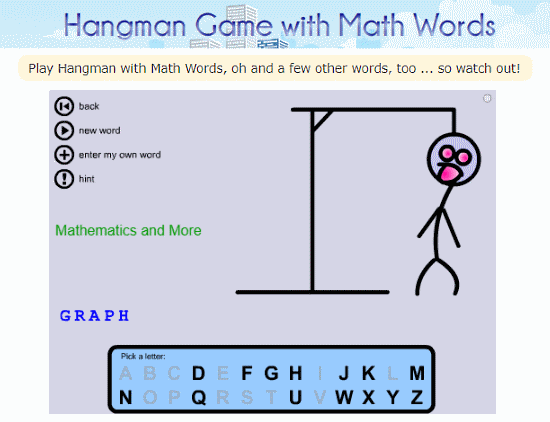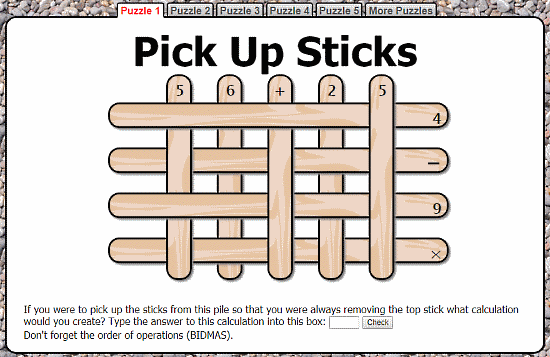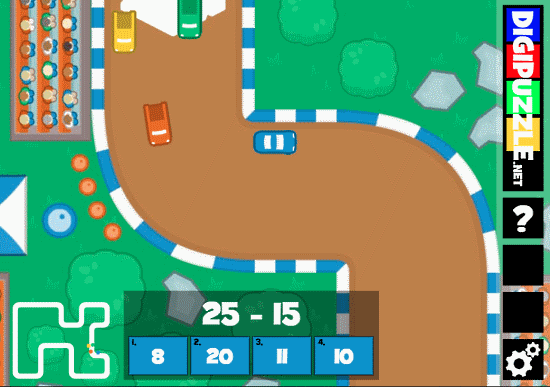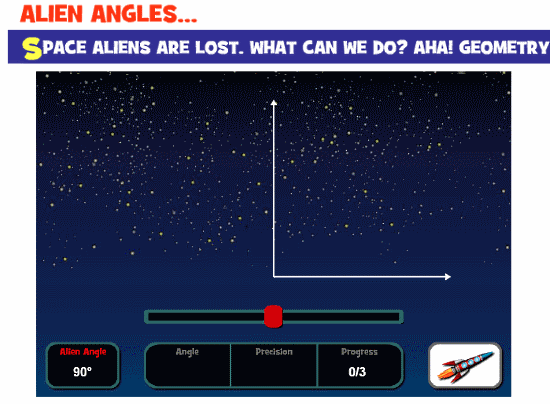Editor Ratings:
User Ratings:
[Total: 0 Average: 0]

In this article, I will cover 5 free websites with online math puzzle games.

These websites offer free math puzzle games that you can play for free. You can find a basic math calculation game to challenging math puzzle games on these websites. Many of these games are heavily influenced by other popular games with the implementation of math.

Some of these websites creatively implemented various math questions and logic in their games. Those creative games are very entertaining and help you improve your math skills at the same time.

## Math Is FunMath Is Fun is a free website that provides various easy and enjoyable ways to learn math. This website has a ‘Puzzle Games‘ section where it offers lots of math-based puzzle games. The one game I really like here is this “Hangman Game With Math Words” (attached screenshot). In this game, you have to guess a math word to save this little fella from hanging. It does not give you any word to begin with; you have to start the guessing from the beginning. Each wrong guess builds one part of the hanging pole taking the fella one step closer to the death.

Apart from this one, there are lots of fun and entertaining games here which you would really enjoy.

Try these online math puzzle games here.

## TransumTransum provides free mathematical activities, problems, visual aids, puzzles and more. It has a dedicated section of math-based puzzles where you can find lots of math puzzle games. Here, you can play a variety of games dealing with shapes, numbers, and various math logic and concepts. For example, this “Pick Up Sticks” game featured in the attached screenshot deals with numbers and logic. It gives you a specific situation, you have to create a calculation to satisfy that situation. If you want to try more fun and entertaining games, you can browse and select the one you find interesting from the collection of 90+ math puzzle games here.

You can try these math puzzle games online here.

## Mind GamesMind Games has a huge collection of puzzle games categories in the 6 sections, Brain Games, Math Games, Puzzle Games, Word Games, Sudoku Games and Memory Games. The Math Games section here features around 30 unique games. You can find a basic math calculation game to challenging math puzzle game here. All these games cover various math logic and rules. For example, the attached screenshot is from a game called “Supermarket Numbers“. At first glance, this game looks very similar to Tetris; it has numbers instead of blocks. Here, you have to match the Goal Number by combining any desired number.

If you like puzzle games that involve calculation then, this is the website for you.

Give this online math puzzle games a try here.

## Digi PuzzleThe next math puzzle games website on this list is Digi PuzzleIt is a free website that helps you learn math in fun and entertaining way. It features lots of puzzle games to help you practice various types of math problems. These games are based on simple math but the way they are implemented is very creative. By looking at the attached screenshot, you might think, it a simple car race game. In that case, you would be partially correct. Yes, it a car game but, it’s not simple. This is a multiplayer game where you play aganist three computer-operated players. Instead of steering and accelerating, you have to solve the math puzzle to keep your car moving. Each correct answer gives your car a boost. Quickly you solve these puzzles, faster your car moves.

All games features on this website are as creative as this one. They are fun, entertaining and help you practice math at the same time.

Check out these creative math puzzle games here.

## Math PlaygroundMath Playground is a website filled with math games, logic puzzles, and other activities. It has hundreds of math-based games implemented in various unique ways. You can find a game for almost every major math topic here. For example, there is this game called “ALIEN ANGLES…“, it helps you practice different angles so, you can easily tell the rough difference between various angles without the need of any apparatus.

There are lots of other learning and entertaining math puzzle games there which you can play and practice math.

Here is a link to try these math puzzle games online.

### Closing Words

All these math puzzle games are really fun and entertaining. many of these games help you practice various math topics thus, help you improve your math. Go, give them a try and put your mind up for a challenge.

 Editor Ratings: User Ratings:[Total: 0 Average: 0] Free/Paid: Free